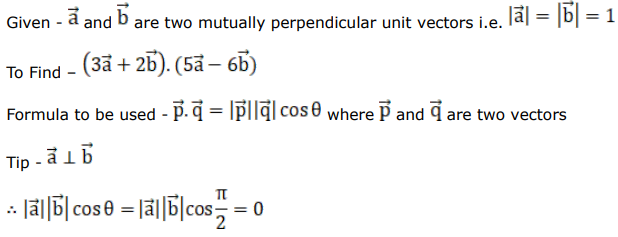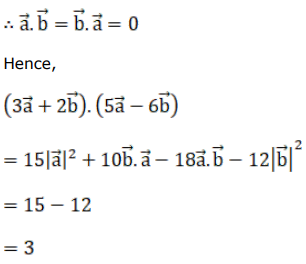# Mark against the correct answer in each of the following:

Question:

Mark $(\sqrt{)}$ against the correct answer in each of the following:

If $\vec{a}$ and $\vec{b}$ are mutually perpendicular unit vectors then $(3 \vec{a}+2 \vec{b}) \cdot(5 \vec{a}-6 \vec{b})=?$

A. 3

B. 5

C. 6

D. 12

Solution: